# wudaijun's blog

Done is better than perfect

### 一. 逻辑回归模型

#### 损失函数和代价函数

$J(w,b)=\frac{1}{m}\sum_1^mL(\hat{y}^{(i)},y^{(i)})=-\frac{1}{m}\sum_1^m[y^{(i)}\lg\hat{y}^{(i)}+(1-y^{(i)})\lg(1-\hat{y}^{(i)})]$

#### 梯度下降模型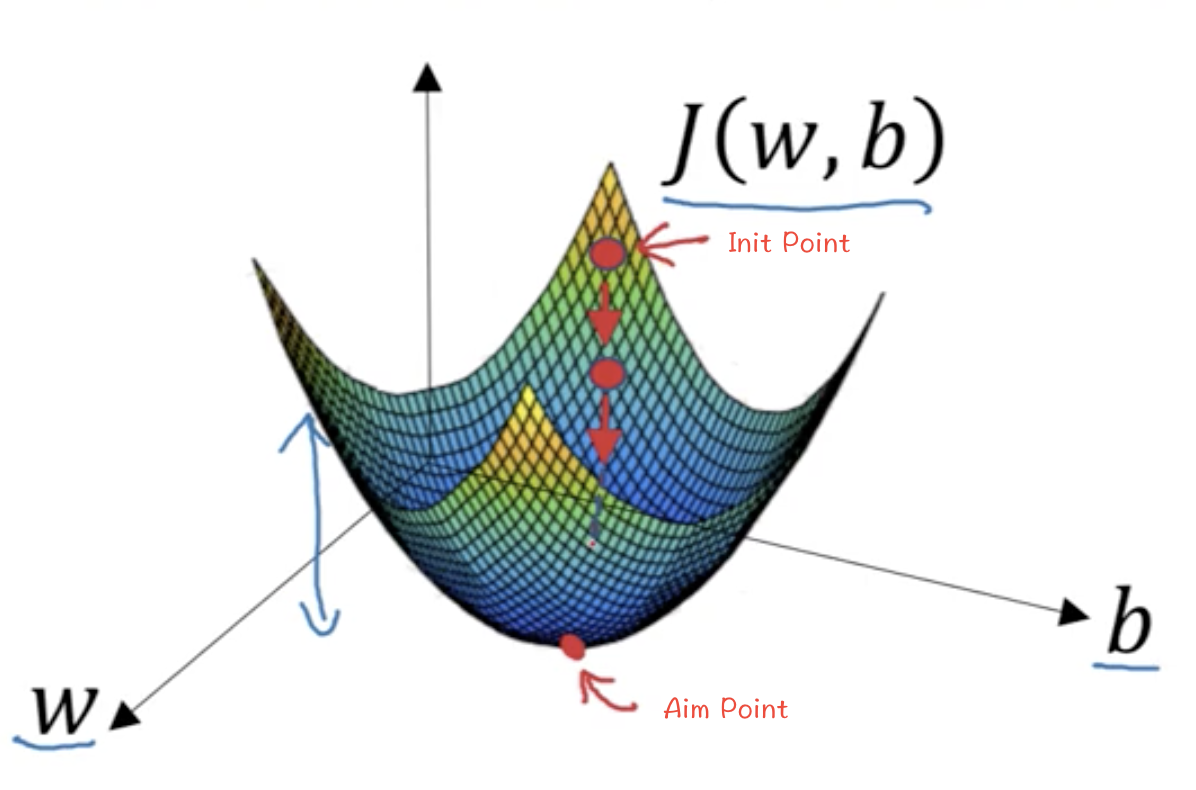$w = w - \alpha * \frac{dJ(w,b)}{dw}$

$b = b - \alpha * \frac{dJ(w,b)}{db}$

#### 对逻辑回归使用梯度下降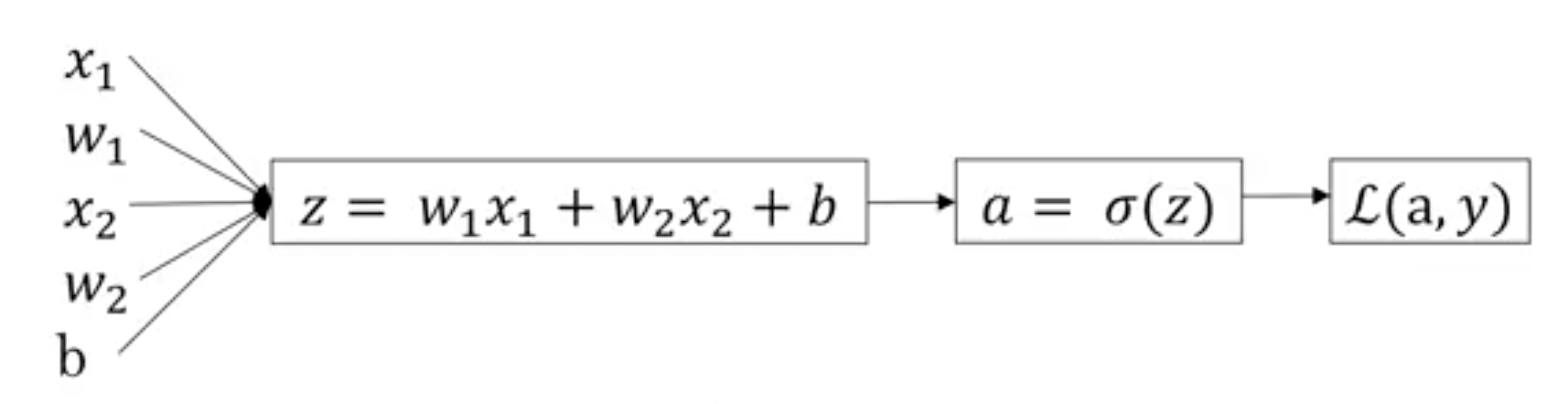$da = \frac{dL}{da} = -\frac{y}{a}+\frac{1-y}{1-a}$

$dz = \frac{dL}{dz} = \frac{da}{dz} * \frac{dL(a,y)}{da} = a(1-a) * (-\frac{y}{a}+\frac{1-y}{1-a}) = a - y$

$dw_1 = \frac{dL}{dw_1} = \frac{dz}{dw_1} * \frac{dL}{dz} = x_1*dz = x_1(a-y)$

$dw_2 = x_2dz = x_2(a-y)$

$db = dz = a-y$

J=0; $dw_1$=0; $dw_2$=0; $db$=0;

for i=1 to m:

$z^{(i)}$ = $w.T*x^{(i)}+b$

$a^{(i)}$ = $\sigma(z^{(i)})$

$J$ += $-[y^{(i)}\lg\hat{y}^{(i)}+(1-y^{(i)})\lg(1-\hat{y}^{(i)})]$

$dz^{(i)}$ = $a^{(i)} - y^{(i)}$

$dw_1$ += $x_1*dz^{(i)}$

$dw_2$ += $x_2*dz^{(i)}$

$db$ += $dz^{(i)}$

end

J /= m; $dw_1$ /= m; $dw_2$ /= m; $db$ /= m

$w_1$ = $w_1$ - $\alpha dw_1$

$w_2$ = $w_2$ - $\alpha dw_2$

$b$ = $b$ - $\alpha db$

1. 用于迭代迭代训练数据个数m的for循环
2. 用于求$dw_1$，$dw_2$…$dw_n$的循环，上面的例子中n=2
3. 用于迭代梯度下降的for循环，这是最外层的for循环

#### 张量计算加速

Z = $w^TX+b$ = np.dot(w.T,X)+b
A = $\sigma(Z)$
dZ = A-Y
dw = $\frac{1}{m}$XdZ.T = np.dot(X, dZ.T)/m
db = $\frac{1}{m}$np.sum(dZ)

### 二. 神经网络

#### 浅层神经网络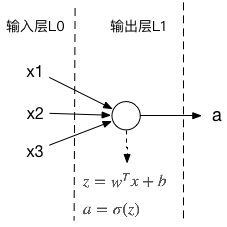##### 正向传播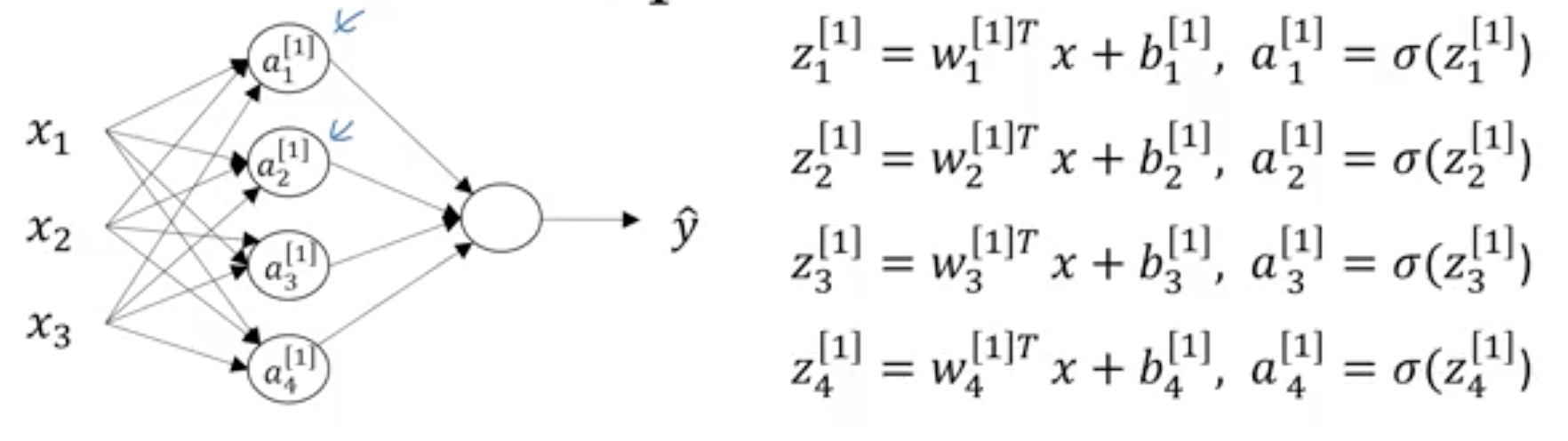$z^{(i)}=w^{T}*x^{(i)}+b^{}$
$a^{(i)}=g^{}(z^{(i)})$

$z^{(i)}=w^{(i)T}*a^{(i)}+b^{(i)}$
$a^{(i)}=g^{}(z^{(i)})$

$Z^{}=W^{}*X+b^{}$
$A^{}=g^{}(Z^{})$
$Z^{}=W^{}*A^{}+b^{}$
$A^{}=g^{}(Z^{})$
$\hat {Y} = A^{}$

##### 反向传播

$g^{} = tanh(z) = \frac{e^z-e^{-z}}{e^z+e^{-z}}$     注: $g^{}\prime = 1-a^2$
$g^{} = \sigma(z) = \frac{1}{(1+e^{-z})}$      注: $g^{}\prime = a(1-a)$

$dZ^{}$ = $A^{} - Y$
$dW^{}$ = $\frac{1}{m}dZ^{}A^{T}$
$db^{}$ = $\frac{1}{m}$np.sum($dZ^{}$)
$dZ^{}$ = $W^{}dZ^{}*1-A^{2}））$
$dW^{}$ = $\frac{1}{m}dZ^{}X^T$
$db^{}$ = $\frac{1}{m}$np.sum(dZ^{})

#### 深度神经网络

##### 正向传播和反向传播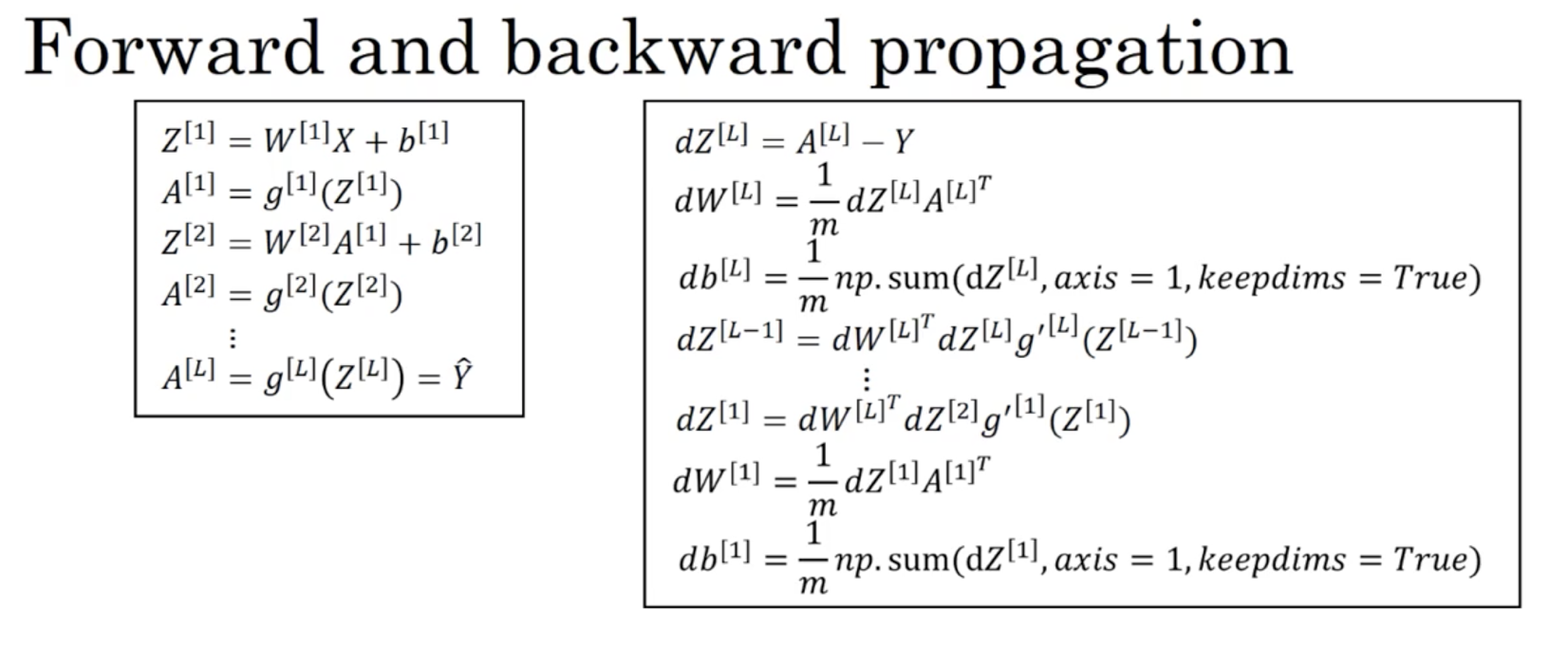$Z^{[l]} = W^{[l]}A^{[l-1]} + b^{[l]}$
$A^{[l]} = g^{[l]}(Z^{[l]})$

$dZ^{[l]} = dA^{[l]}*g^{[l]}\prime(Z^{[l]})$
$dW^{[l]} = \frac{1}{m}dZ^{[l]}*A^{[l-1]T}$
$db^{[l]} = \frac{1}{m}$np.sum($dZ^{[l]}$)
$dA^{[l-1]} = W^{[l]T}*dZ^{[L]}$

##### 参数和超参数

1. 学习率/步长 $\alpha$
2. 迭代次数
3. 神经网络的层数L
4. 每层的神经元数$n^{[l]}$
5. 每层激活函数的选择